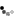Loading... Please wait...

Previous Page

Smalti Area Calculator

Select the shape you want to calculate. Then enter the dimensions in inches and click the Calculate Area button. Depending upon your project, you may want to add a percent overage to account for cutting and shaping. For most projects, a 10% overage is appropriate.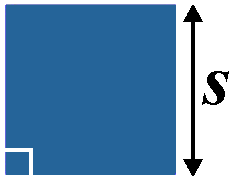Square
Area = s2
s = length of side =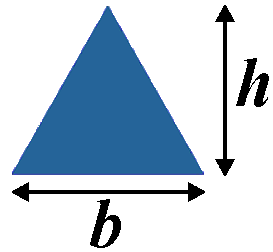Triangle
Area = ½b × h
b = base =
h = vertical height =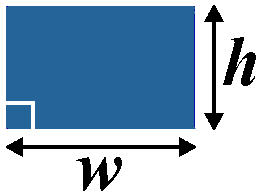Rectangle
Area = w × h
w = width =
h = height =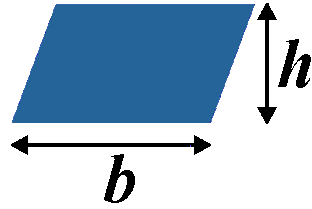Parallelogram
Area = b × h
b = base =
h = vertical height =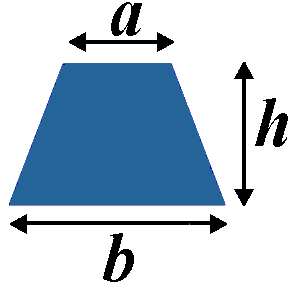Trapezoid
Area = ½(a+b) × h
h = vertical height =
a =   b =Circle
Area = πr2
r = radius =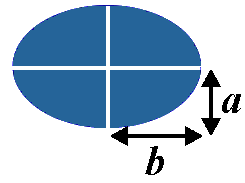Ellipse
Area = πab
a =
b =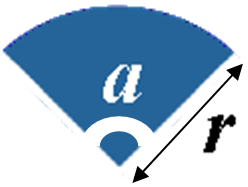Sector
Area = ½r2 x aπ/180
r = radius =
a = angle in degrees =

Percent overage %
 Shape sq in Smalti lbs pieces Stone lbs pieces Gold lbs pieces